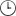You have not yet responded to the forum.

Here you will find the last 3 forum topics
you have posted a comment on.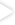To join the forums you need to be logged in.

> Close11 of the 24 stars earned

# Forum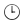Simple math helppp
CoolAsola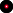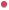International starWhy is 3^10 +3^10+3^10 = 3^11?

Plssss someone give me a rule of this thing
CoolAsolaInternational starSomeone plsPrivate
Minister of Popi didnt kno it was tru so i had to check on calculator lol

but for each number u add to the ^ it tripples right, bc the base is 3

so 3^11 is 3 times 3^10
Private
International starthe rule is addition, three to the tenth power means 3x3x3 etc. until you get to 10 3s. the answer  to 3^10 is 59049 and when you multiply that by three you get 177147. three to the 11th power is the same, 3x3x3 etc. until you have 11 threes. the answer is also 177147
CoolAsolaInternational starI still don't understand😭😭 im so stupid
Thank u guys anyway
<333
Private
International starCoolAsola wrote:
I still don't understand😭😭 im so stupid
Thank u guys anyway
<333
its okay, which part don't you understand?
CoolAsolaInternational starDracarys wrote:
CoolAsola wrote:
I still don't understand😭😭 im so stupid
Thank u guys anyway
<333
its okay, which part don't you understand?
Why did u multipy it by 3 after ubfound what 3^10 is?
Is there any easier way bc that one is p hard to calculate in 1 min without a calculator 😭
Private
International starCoolAsola wrote:
Dracarys wrote:
CoolAsola wrote:
I still don't understand😭😭 im so stupid
Thank u guys anyway
<333
its okay, which part don't you understand?
Why did u multipy it by 3 after ubfound what 3^10 is?
Is there any easier way bc that one is p hard to calculate in 1 min without a calculator 😭
It's just another way to add 3^10+3^10+3^10.

3^10     +  3^10    +  3^10
59049  + 59049 +  59049 = 177147

Or
3^10
59049 multiplied by 3

not really. you'll only have one minute?Private
Living legendHuuuuh
Fiexi'm so glad i'm done with maths
CoolAsolaInternational starDracarys wrote:
CoolAsola wrote:
Dracarys wrote:
its okay, which part don't you understand?
Why did u multipy it by 3 after ubfound what 3^10 is?
Is there any easier way bc that one is p hard to calculate in 1 min without a calculator 😭
It's just another way to add 3^10+3^10+3^10.

3^10     +  3^10    +  3^10
59049  + 59049 +  59049 = 177147

Or
3^10
59049 multiplied by 3

not really. you'll only have one minute?
Okaaaay i get it now. Thanks ALOT.❤️❤️
Yes unfortunately. I dont know how will i manage to do these calculations without a calculator. & on top of that i forgot all the multiplication timetable lol
CoolAsolaInternational starFiex wrote:
i'm so glad i'm done with maths
Im waiting for that day ahh
Post comment
Post Comment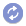To load new posts: activated